## Wednesday, May 9, 2007

### Video Fundamentals

1.1
Introduction

Video is an illusion that makes use of the properties of eye. Our eye has a peculiar property that image sensed by eye persists forth of a second: ie our eye cannot notice the change of scene in this period. It’s called persistence of vision. We can make the illusion of continuity of a scene by capturing just more than 16 pictures of the visual in a second and displaying the same on a screen in the same time period. It’s the basic principle behind video rendering. Each picture captured is called frames. Therefore a frame rate more than 16 is convenient. Usually we go for 25 fps or more. In the earlier television system we get the image by projecting an electron beam over a phosphor screen. The phosphor screen is divided into 525 lines in case PAL TV system or 625 lines in the case of NTSC system. The electron beam sequentially scans the 525/625 lines from left to right.Figure 2.1 Progressive scan

When the electron beams, intensity modulated with the video signal, incidents on the phosphor screen, it starts glowing. The intensity of glow depends on the strength of video signal. The way of scanning in the sequential mode is called Progressive scan (Figure 2.1).

In the case of Progressive scanning, there is a finite delay, 0.04s, between successive frames.The point on the phosphor screen corresponding to an image should glow for that much time. But usually the screen has a poor response and results in flicker: ie the image fades and screen tends to become black. An ideal solution to remove the flickering problem is to increase the frame rate. But it will result in the increased bandwidth usage as the amount of data corresponding to those frames increases. Another solution for this problem is the interlaced scanning. A frame is divided into two fields, odd and even. Odd field is obtained by grouping the odd lines in the frame together and even field are obtained by grouping the even lines in the frame. Scanning the fields at different instant avoids the problem of flickering to an extent and doesn’t introduce burden of bandwidth hike. The screen refresh gets doubled. This type of scanning is called interlaced scanning, illustrated in Figure 2.2.

Figure 2.2 Interlaced scan

## 1.2 Video Coding Concepts

Data compression is achieved by removing redundancy, i.e. components that are not necessary for faithful reproduction of the data. Many types of data contain statistical redundancy and can be effectively compressed using lossless compression, so that the reconstructed data at the output of the decoder is a perfect copy of the original data. Unfortunately, lossless compression of image and video information gives only a moderate amount of compression. The best that can be achieved with current lossless image compression standards is a compression ratio of around 3–4 times. Lossy compression is necessary to achieve higher compression. In a lossy compression system, the decompressed data is not identical to the source data and much higher compression ratios can be achieved at the expense of a loss of visual quality. Lossy video compression systems are based on the principle of removing subjective redundancy, elements of the image or video sequence that can be removed without significantly affecting the viewer’s perception of visual quality. Compression results in higher computational complexities.

Most video coding methods exploit both temporal and spatial redundancy to achieve compression. In the temporal domain, there is usually a high correlation (similarity) between frames of video that are captured at around the same time, especially if the temporal sampling rate (the frame rate) is high. In the spatial domain,

there is usually a high correlation between pixels (samples) that are close to each other, i.e. the values of neighboring samples are often very similar. Spatial and temporal redundancy are illustrated in the Figure 2.3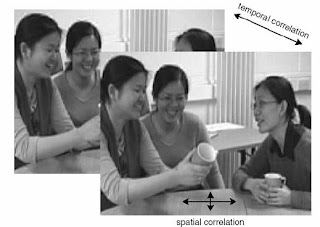Figure 2.3 Spatial & temporal correlation in a video sequence

## 1.3 Spatial Model

Due to the temporal correlation of frames, successive frames can be predicted from the previous one. For this we need reference frames. Reference frames are encoded in a very similar fashion as explained in section 1.5.

## 1.4 Temporal Model

The goal of the temporal model is to reduce redundancy between transmitted frames by forming a predicted frame and subtracting this from the current frame. The output of this process is a residual (difference) frame. The more accurate the prediction process, the

Figure 2.4 Difference of frame-1 and frame-2

less energy is contained in the residual frame. The residual frame is encoded

and sent to the decoder which re-creates the predicted frame, adds the decoded residual and reconstructs the current frame. The predicted frame is created from one or more past or future frames (‘reference frames’). The accuracy of the prediction can usually be improved by compensating for motion between the reference frame(s) and the current frame.

The simplest method of temporal prediction is to use the previous frame as the predictor for the current frame. Two successive frames from a video sequence are shown in Frame 1 and Frame 2 in Figure 2.4.. Frame 1 is used as a predictor for frame 2 and the residual formed by subtracting the predictor (frame 1) from the current frame (frame 2) is shown in Figure 2.4. The obvious problem with this simple prediction is that a lot of energy remains in the residual frame (indicated by the light and dark areas) and this means that there is still a significant amount of information to compress after temporal prediction. Much of the residual energy is due to object movements between the two frames and a better prediction may be formed by compensating for motion between the two frames.

## 1.5 Optic Flow

Changes between video frames may be caused by o

bject motion (rigid object motion, for

##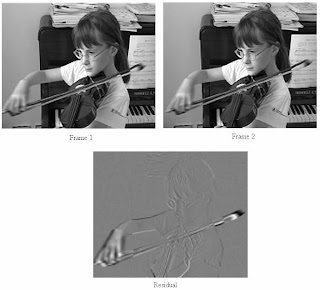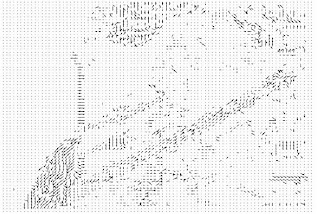Figure 2.5 Optical Flow

example a moving car, and deformable object motion, for example a moving arm), camera motion (panning, tilt, zoom, rotation), uncovered regions (for example, a portion of the scene background uncovered by a moving object) and lighting changes. With the exception of uncovered regions and lighting changes, these differences correspond to pixel movements between frames. It is possible to estimate the trajectory of each pixel between successive video frames, producing a field of pixel trajectories known as the optical flow (optic flow). Figure 2.5 shows the optical flowfield for the frames 1 and 2 of Figure 2.4. The complete field contains a flow vector for every pixel position but for clarity, the field is sub-sampled so that only the vector for every 2nd pixel is shown.

If the optical flow field is accurately known, it should be possible to form an accurate prediction of most of the pixels of the current frame by moving each pixel from the reference frame along its optical flow vector. However, this is not a practical method of motion compensation for several reasons. An accurate calculation of optical flow is very computationally intensive (the more accurate methods use an iterative procedure for every pixel) and it would be necessary to send the optical flow vector for every pixel to the decoder.

## 1.6 Block-based Motion Estimation and Compensation

A practical and widely-used method of motion compensation is to compensate for movement of rectangular sections or ‘blocks’ of the current frame. The following procedure is carried out for each block of M × N samples in the current frame:

1. Search an area in the reference frame (past or future frame, previously coded and transmitted) to find a ‘matching’ M × N-sample region. This is carried out by comparing the M × N block in the current frame with some or all of the possible M × N regions in the search area (usually a region centered on the current block position) and finding the region that gives the ‘best’ match. A popular matching criterion is the energy in the residual formed by subtracting the candidate region from the current M × N block, so that the candidate region that minimizes the residual energy is chosen as the best match. This process of finding the best match is known as motion estimation.

2. The chosen candidate region becomes the predictor for the current M × N block and is subtracted from the current block to form a residual M × N block (motion compensation, MC).

3. The residual block is encoded and transmitted and the offset between the current block and the position of the candidate region (motion vector, MV) is also transmitted. The decoder uses the received motion vector to re-create the predictor region and decodes the residual block, adds it to the predictor and reconstructs a version of the original block.

Block-based motion compensation is popular for a number of reasons. It is relatively straight forward and computationally tractable, it fits well with rectangular video frames and with block-based image transforms (e.g. the Discrete Cosine Transform, see later) and it provides a reasonably effective temporal model for many video sequences. There are however a number of disadvantages, for example ‘real’ objects rarely have neat edges that match rectangular boundaries, objects often move by a fractional number of pixel positions between frames and many types of object motion are hard to compensate for using block-based methods (e.g. deformable objects, rotation and warping, complex motion such as a cloud of smoke). Despite these disadvantages, block-based motion compensation is the basis of the temporal model used by all current video coding standards.

#### 1.6.1.1 Motion Compensated Prediction of Macroblock

Image is divided into macroblocks (MB) of size N × N. By default, N = 16 for luminance images. For chrominance images N = 8 if 4:2:0 chroma subsampling (explained in section 1.6.2) is adopted. H.264 uses a variant of this. Motion compensation is performed at the macroblock level. Macroblock partitioning is shown in Figure 2.6. The general rule of prediction is as follows:

- The current image frame is referred to as target frame.

- A match (only for Y macroblock) is sought between the macroblock in the Target Frame and the most similar macroblock in previous and/or future frame(s) (referred to as reference frame(s)).

- The displacement of the reference macroblock to the target macroblock is called a motion vector, MV.

- Residual is found in case of Y macroblock as well as Cb & Cr macroblocks.

- Figure 2.7 shows the case of forward prediction in which the reference

frame is taken to be a previous frame.

- MV search is usually limited to a small immediate neighborhood - both

horizontal and vertical displacements in the range [−p, p]. This makes a search window of size (2p + 1) × (2p + 1).Figure 2.6 Size of macroblock for prediction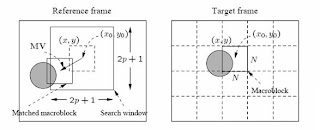Figure 2.7 Motion Compensated prediction of Macroblock

General Rule for searching motion vectors

Parameter MAD (Mean Absolute Differnce) is defined as

MAD (i, j) =| C(x + k, y + l) – R (x + i + k, y + j + l)| eqn 2.1

where

N - size of the macroblock,

k and l - indices for pixels in the macroblock,

i and j - horizontal and vertical displacements,

C(x + k, y + l) - pixels in macroblock in Target frame,

R(x + i + k, y + j + l) - pixels in macroblock in Reference frame

The search is to find a vector (i, j) as the motion vector MV = (u, v), such that MAD(i, j) is minimum:

(u, v) = { (i, j) | MAD(i, j) is minimum, i ε [−p, p]; j ε [−p; p] }

### 1.6.2 Frame Coding

RAW video can be viewed as a sequence of frames in the display rate of 25 frames per second or above. Encoder converts these frames as Intra-frames (I-frames), Inter-frames (P-frames) or Bidirectional frames (B-frames). Transform coding method similar to JPEG is applied within each I-frame, hence the name “Intra”. P-frames are not independent, coded by a forward predictive coding method (prediction from a previous P-frame is allowed - not just from a previous I-frame). B frame is predicted from more than one frame usually from a previous and a future frame.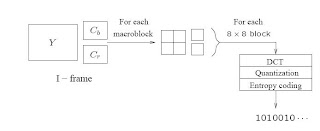Figure 2.8 I-frame Coding

I-frame coding is depicted in figure 2.8. Here Macroblocks are of size 16 × 16 pixels for the Y frame, and 8 × 8 for Cb and Cr frames, since 4:2:0 chroma subsampling is apllied. A macroblock consists of four Y, one Cb, and one Cr 8 × 8 blocks. For each 8 × 8 block a DCT transform is applied, the DCT coefficients then go through quantization zigzag scan and entropy coding.

P-frame coding is based on Motion Compensation. For each macroblock in the Target frame, a motion vector is allocated. After the prediction, a difference macroblock is derived to measure the prediction error. Each of these 8 × 8 blocks go through DCT, quantization, zigzag scan and entropy coding procedures. The P-frame coding encodes the difference macroblock (not the Target macroblock itself). Sometimes, a good match cannot be found, i.e., the prediction error exceeds a certain acceptable level. The MB itself is then encoded (treated as an Intra MB) and in this case it is termed a non-motion compensated MB. For motion vector, the difference MVD is sent for entropy coding. Figure 2.9 gives an overview on P-frame coding.

Figure 2.9 P-frame coding

Motion Compensation based B-frame coding method is illustrated in Figure. 2.10.

Each Macroblock from a B-frame will have up to two motion vectors (MV’s) (one from the forward and one from the backward prediction). If its matching in both directions, then two MVs will be sent and the two corresponding matching MBs are averaged (indicated by `%' in the figure 2.10) before comparing to the Target MB for generating the prediction error. If an acceptable match can be found in only one of the reference frames, then only one MV and its corresponding MB will be used from either the forward or backward prediction.

Figure 2.10 B-frame CodingFigure 2.11 4:2:0 Subsampling in the case of interlaced video

### 1.6.3 Field Prediction

Field prediction comes in the case of codecs having an extended support to interlaced video. Chroma subsampling in the case of interlaced video is illustrated in the figure 2.11.In interlaced video each frame consists of two fields, referred to as the top-field and the bottom-field. In a frame-picture, all scanlines from both fields are interleaved to form a single frame, then divided into 16 x 16 macroblocks and coded using motion compensation. If each field is treated as a separate picture, then it is called field picture.

Figure 2.12 Frame picture Vs Field picture

The common modes of prediction in case of interlaced video:
1) Frame Prediction for Frame-pictures

Fields are combined to form frames and prediction is performed for macro blocks of size 16 × 16, in a similar fashion explained as in 2.4.3.

2) Field Prediction for Field-pictures

A macroblock size of 16 × 16 from field pictures is used, as in figure 2.13Figure 2.13 Field Prediction for field pictures

3) Field Prediction for Frame-pictures

The top field and bottom field of a frame picture are treated separately. Each

16 x 16 macroblock (MB) from the target frame picture is split into two 16 x 8 parts, each coming from one field. Field prediction is carried out for these 16 x 8 parts in a manner shown in Figure 2.14

Figure 2.14 Field Prediction for frame-pictures

Each 16 x16 macroblock (MB) from the target field picture is split into top and

bottom 16 x 8 halves. Field prediction is performed on each half. This generates two motion vectors for each 16 x16 MB in the P-field picture, and up to four motion vectors for each MB in the B-field picture. This mode is good for a finer MC when motion is rapid and irregular. Figure 2.15 gives an illustration.Figure 2.15 16 x 8 motion compensation for field-pictures.

5) Dual-Prime for P-pictures

Field prediction from each previous field with the same parity (top or bottom) is made. Each motion vector MV is then used to derive a calculated motion vector CV in the field with the opposite parity taking into account the temporal scaling and vertical shift between lines in the top and bottom fields. For each MB the pair MV and CV yields two preliminary predictions. Their prediction errors are averaged and used as the final prediction error. This mode mimics B-picture prediction for P-pictures without adopting backward prediction (and hence with less encoding delay). This is the only mode that can be used for both frame pictures and field-pictures.

### 1.6.4 Transform Coding

In case of I-frames, the 8 × 8 blocks of Y, Cb and Cr are transform coded. And in case of B-frames and P-frames the residual value obtained from block based motion estimation is transform coded. Figure 2.16 shows the 8 × 8 block division of a residual macroblock for the luma signal in the case of interlaced video. These 8 × 8 blocks are transform coded in a similar manner as in section 1.5.4. Similar is the case for chroma signal.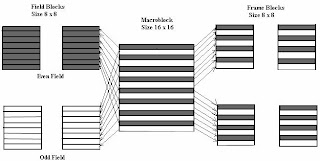Figure 2.16 Frame and field DCT for a Y macroblock in interlaced video

### 1.6.5 Quantization

In the case video coding two quantization tables are used, one for intra-coding and the other for inter-coding in the case of MPEG-1 & MPEG-2. Also another parameter scale called quantization parameter (QP) is defined to use the variants of quantization matrix.

Zij = Round () eqn 2.2

Zij = Round () eqn 2.3

Where Z is the quantized matrix and QP, quantization parameter and Q1, Q2 represents the quantization matix for intra and inter coding respectively.

The value of QP varies from 1 – 31 and in effect we have more no number of quantization table. The wide range of quantizer step sizes makes it possible for an encoder to control the tradeoff accurately and flexibly between bit rate and quality.

Table 2.1 The matrix used for intra coding for eqn 1.1

Table 2.2 The matrix used for inter coding for eqn 1.2

### 1.6.6 Zig-Zag ScanTwo different types of reordering for the Transform coded values are found, zig-zag Figure 2.17 Reordering of quantized transform coefficients.

found, zig-zag scan for progressive video and another alternate scan for interlaced

video shown in figure 2.17. Refer section 1.5.6.

### 1.6.7 Run Level Coding

The output of the reordering process is an array that typically contains one or more clusters of nonzero coefficients near the start, followed by strings of zero coefficients. The large numbers of zero values are encoded to represent them more compactly, for example by representing the array as a series of (run, level) pairs where run indicates the number of zeros preceding a nonzero coefficient and level indicates the signed magnitude of the nonzero coefficient. Higher-frequency DCT coefficients are very often quantized to zero and so a reordered block will usually end in a run of zeros. A special case is required to indicate the final nonzero coefficient in a block, EOB (End of Block). This is called ‘Two-dimensional’ run-level encoding. If ‘Three-dimensional’ run-level encoding is used, each symbol encodes three quantities, run, level and last. Last indicates whether the level is the last non zero value in the linear array.

Example:

Input array (Zig Zag ordered value) : 16,0,0,−3,5,6,0,0,0,0,−7,0,0 . . .

2D Coding:

Output values : (0, 16), (2, −3), (0, 5), (0, 6), (4, −7), (EOB)

Each of these output values (a run-level pair) is encoded as a separate symbol by the entropy encoder.

3D coded values are:

Output values :(0, 16, 0), (2,−3, 0), (0, 5, 0), (0, 6, 0), (4,−7, 1)

The 1 in the final code indicates that this is the last nonzero coefficient in the block

### 1.6.8 Entropy Encoding

The entropy encoder converts a series of symbols representing elements of the video sequence into a compressed bitstream suitable for transmission and storage. In this section we discuss the widely-used entropy coding techniques.

#### 1.6.8.1 Variable-length Coding

A variable-length encoder maps input symbols to a series of codewords (variable length codes or VLCs). Each codeword may have varying length but each must contain an integral number of bits. Frequently occurring symbols are represented with short VLCs while less common symbols are represented with long VLCs. Over a sufficiently large number of encoded symbols this leads to compression of the data.

#### 1.6.8.2 Huffman Coding

Huffman coding assigns a VLC to each symbol based on its probability of occurrence. According to the original scheme proposed by Huffman, it is necessary to calculate the probability of occurrence of each symbol and to construct a set of variable length codewords. Based on this key word we can encode and decode symbols. Since the VLC formed by Huffman algorithm is a prefix code we can easily decode the same to corresponding symbol.

Consider the probability distribution of MVD (Motion Vector Differences) given in table 2.3.

 Vector Probability Code 0 0.8 1 1 0.08 01 -1 0.07 001 2 0.03 0001 -2 0.02 0000

Table 2.3 Code word for vector, based on Huffman Algorithm

If vector sequence is (1, -2, 0, 0, 2), then its binary sequence is 010000110001. In order to decode the data, the decoder alsomust have a copy of the Huffman code tree (or look-up table). This may be achieved by transmitting the look-up table itself or by sending the list of data and probabilities prior to sending the coded data. Each uniquely-decodeable code is converted back to the original data, for example:

01 is decoded as (1)

0000 is decoded as (-2)

1 is decoded as (0).

1 is decoded as (0).

0001 is decoded as (2).

#### 1.6.8.3 Pre-calculated Huffman-based Coding

The Huffman coding process has two disadvantages for a practical video CODEC. First, the decoder must use the same codeword set as the encoder. Transmitting the informationTable 2.4 Pre-calculated Huffman table used for MPEG-4 Coding

contained in the probability table to the decoder would add extra overhead and reduce compression efficiency, particularly for shorter video sequences. Second, the probability table for a large video sequence (required to generate the Huffman tree) cannot be calculated until after the video data is encoded which may introduce an unacceptable delay into the encoding process. For these reasons, recent image and video coding standards define sets of codewords based on the probability distributions of ‘generic’ video material. Such a pre-calculated VLC table used by MPEG-4 Visual (Simple Profile) is shown in table 2.4.

#### 1 comment:

1.